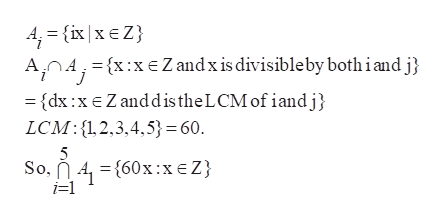Use the definition for Ai to answer the questions.For i ∈ Z+, Ai is the set of all integer multiples of i.(a)Describe the following set using set builder notation:   ⋂i=15Ai(b)Describe the following set using roster notation:          (⋃i=25Ai)∩{x∈Z:1≤x≤20}

Question

Use the definition for Ai to answer the questions.
For i ∈ Z+, Ai is the set of all integer multiples of i.
(a)
Describe the following set using set builder notation:

⋂i=15Ai
(b)
Describe the following set using roster notation:

(⋃i=25Ai)∩{x∈Z:1≤x≤20}

Step 1

Given information:

For i ∈ Z+, Ai is the set of all integer multiples of i.

Step 2

Calculation:

Let us assume that A_i={......, -3i,...help_outlineImage Transcriptionclose4 (ix xE Z AOAx:x Z and x is divisibleby both iand j = {dx:xEZanddistheL CM of iandj LCM:12,3,4,5}=60 5 So, A{60x:xeZ i-1 fullscreen

Want to see the full answer?

See Solution

Want to see this answer and more?

Our solutions are written by experts, many with advanced degrees, and available 24/7

See Solution
Tagged in

Algebra